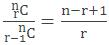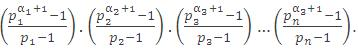Click to Chat

1800-1023-196

+91-120-4616500

CART 0

• 0

MY CART (5)

Use Coupon: CART20 and get 20% off on all online Study Material

ITEM
DETAILS
MRP
DISCOUNT
FINAL PRICE
Total Price: Rs.

There are no items in this cart.
Continue Shopping```Restricted Selection and Arrangement(a) The number of ways in which r objects can be selected form n different objects if k particular objects are

(i) Always included = n-kCr-k.

(ii) Never included = n-kCr.

(b) The number of arrangement of n distinct objects taken r at a time so that k particular objects are

(i) Always included = n-kCr-k.r!,

(ii) Never included = n-kCr.r!.

Illustration:

A delegation of four students is to be selected form a total of 12 students. In how many ways can the delegation be selected if

(a) All the students are equally willing.

(b) Two particular students have to be included in the delegation.

(c) Two particular students do not wish to be together in the delegation.

(d) Two particular students wish to be included together only,

(e) Two particular students refuse to be together and two other particular student wish to be together only in the delegation.

Solution:

(a) Formation of delegation means selection of 4 out of 12. Hence the number of ways = 12C4 = 495.

(b) Two particular students are already selected. Hence we need to select 2 out of the remaining 10. Hence the number of  ways = 10C2 = 45.

(c) The number of ways in which both are selected = 45. Hence the number of ways in which the two are not included together = 495 - 45 = 450.

(d) There are two possible cases

(i) Either both are selected. In this case the number of ways in which this selection can be made = 45.

or

(ii) both are selected. In this case all the four students are selected from the rest of ten students.

This can be done in 10C4 = 210 ways.

Hence the total number of ways of selection = 45 + 210 = 255.

(e) We assume that students A and B wish to be selected together and students C and D do not wish to be together. Now there are following 6 cases.

(i) (A, B, C)           selected             (D) not selected

(ii) (A, B, D)           selected             (C) not selected

(iii) (A, B)              selected             (C, D) not selected

(iv) (C)                 selected             (A, B, D) not selected

(v) (D)                  selected             (A, B, C) not selected

(vi) A, B, C, D        not selected

For (i) the number of ways selection = 8C1 = 8

For (ii) the number of ways selection = 8C1 = 8

For (iii) the number of ways selection = 8C2 = 28

For (iv) the number of ways selection = 8C3 = 56

For (v) the number of ways selection = 8C3 = 56

For (vi) the number of ways selection = 8C4 = 70

Hence, total number of ways = 8 + 8 + 28 + 56 + 56 + 70 = 226.

Some results related to nCr

(i) nCr = nCn-r

(ii) If nCr = nCk, then r = k or n-r = k

(iii) nCr + nCr-1 = n+1Cr

(iv) nCr = n/r  n-1Cr-1

(v)(vi) (a) If n is even nCr is greatest for r = n/2

(b) If n is odd, is greatest for r = (n-1)/2,(n+1)/2

Illustration:

(a) How many diagonals are there in as n-sided polygon (n > 3?

(b) How many triangles can be formed by joining the vertices of an
n-sided polygon?

How many these triangles have

(i) Exactly one side common with that of the polygon?

(ii) Exactly two sides common with that of the polygon?

(iii) No side common with that of the polygon?

Solution:

(a) Number of lines formed by joining the vertices of a polygon

= number of selection of 2 points each selected from the given n points

= nC2 = (n(n-1)!)/2.1 .

Out of nC2 lines, n are the sides of the polygon.

Hence the number of diagonals = nC2-n = ((n(n-1))/2) - n = (n(n-3))/2 .

(b) Number of triangles formed by joining the vertices of the polygon = number of selection of 3 points from n points.

nC3 = (n(n-1)(n-2))/3.2.1 .

Let the vertices of the polygon be marked as A1, A2, A3, ......, An.

(i) Select two consecutive vertices A1, A2 of the polygon. For the required triangles we can select the third vertex from the A4, A5, ..., An-1. This can be done in n-4C1 ways. Also two consecutive points (end points of a side of polygon) can be  selected in n ways.

(ii) For the required triangle, we have to select three consecutive vertices of the polygon. i.e. (A1 A2 A3), (A2 A3 A4) (A3 A4A5) ... (An A1 A2). This can be done in n ways.

(iii) Triangle having no side common + triangle having exactly one side common + triangle having exactly two sides common

(with those of the polygon) = Total number triangles formed

=> Triangle having no side common with those of the polygon.

= nC3 - n(n - 4) - n = ((n(n-1)(n-2))/6) -n(n - 4) - n.

= n/6 [n2 + 3n + 2 - 6n + 24 - 6] =  [n2 + 9 + 20] = (n(n-4)(n-5))/6.

All possible selections

(i) Selection from distinct objects :

The number of selections from n different objects, taking at least one = nC1 + nC2 + nC3 + ... + nCn = 2n - 1.

In other words, for every object we have two choices, either select or reject in a particular group. Total number of choice (all possible selections) = 2.2.2. ...n times = 2n. But this also includes the case when none is selected. And the number of such cases is 2n - 1.

(ii) Selection from identical objects:

(a) The number of selections of r objects out of n identical objects is 1.

(b) Total number of selections of zero or more objects from n identical objects is n+1.

(c) The total number of selections of at least one out of a1 + a2 + a3 + ...... + an objects, where a1 are alike (of one kind), a2 are alike (of second kind) and so on ...... an are alike (of nth kind), is

[(a1 + 1)(a2 + 1)(a3 + 1).........(an + 1)] - 1.

(iii) Selection when both identical and distinct objects are present:

The number of selections, taking at least one out of a1 + a2 + a3 + ... an + k objects, where a1 are alike (of one kind), a2 are alike (of second kind) and so on ... an are alike (of nth kind), and k are distinct =
{[(a1 + 1)(a2 + 1)(a3 + 1) ... (an + 1)]2k} - 1.

Illustration:

In how many ways can a person having 3 coins of 25 paise, 4 coins of 50 paise and 2 coins of 1 rupee give none or some coins to a beggar?

Solution:

The person has 3 coins of 25 paise, 4 coins of 50 paise and 2 coins of 1 rupee.

The number of ways in which he can give none or some coins to a beggar is (3 + 1)(4 + 1)(2 + 1) = 60 ways.

(iv) (a) Total number of divisor's of a given natural number

To find the number of factors of a given natural number greater than 1 we can write n as n =, where p1, p2, ......, pn are distinct prime numbers and α1, α2 ...... αn are non-negative integers. Now any divisor of n will be of the form d =; here number of factors will be equal to numbers of ways in which we can choose β1s' which can be done in (α1 + 1)(α2 + 1)......(αn + 1) ways.

Sum of all the divisors of n is given by(b) The exponent of a prime number p1 in n =is given byIllustration:

How many positive factors are there of the number 360 and find the sum of all these factors.

Solution:

Let n = 360 = 23.32.5

=> No. of factors of 360 = (3 + 1)(2 + 1)(1 + 1) = 24.

Sum of all the factors =((24-1)/1) . ((32-1)/2) . ((52-1)/4) = 15. 13. 6 = 1170.

AskIITians offers a novel way of teaching where you can prepare for IIT JEE, AIEEE and other engineering examinations for free by sitting at home. You can visit the website askIITians.com to read the study material pertaining to your preparation. Be a part of our online tests and AQAD (A Question A Day) for free and be a winner.

To read more, Buy study materials of Permutations and Combinations comprising study notes, revision notes, video lectures, previous year solved questions etc. Also browse for more study materials on Mathematics here.
```### Course Features

• 731 Video Lectures
• Revision Notes
• Previous Year Papers
• Mind Map
• Study Planner
• NCERT Solutions
• Discussion Forum
• Test paper with Video Solution